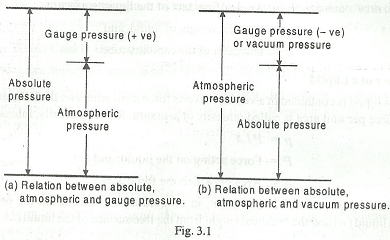Showing posts with label Hydraulics and Fluid Mechanics. Show all posts
Showing posts with label Hydraulics and Fluid Mechanics. Show all posts

### Hydraulics and Fluid Mechanics

Basic concepts of Hydraulics and Fluid Mechanics course with all fundamentals including properties of liquid, pascal's law, fluid kinematics, equation of continuity, Bernoulli's equation and viscous flows. Below is complete outline of the subject as taught in mechanical engineering undergraduate course. It is compulsory for all mechanical engineers to revise basics of hydraulics and fluid mechanics and other courses to stay updated with knowledge of the subjects and for a better performance during interviews. It has been learnt that many interviewers like to ask basic questions from fluid mechanics during interview. Once you have gone through all the short topics given below, you will be in a position to answer all interview questions related to hydraulics/ fluid mechanics.

### Hydraulics and Fluid Mechanics - Introduction

The word 'Hydraulics' has been derived from a Greek word 'Hudour' which means water. The subject 'Hydraulics' is that branch of Engineering - science which deals with water at rest or in motion. The subject 'Fluid Mechanics' is that branch of Engineering-science which deals with the behavior of fluid under the conditions of rest and motion.

### Important Terms Used in Hydraulics and Fluid Mechanics

Density or mass density, Weight density or specific weight, specific volume and specific gravity are important terms used in Hydraulics and Fluid Mechanics :

I. Density or mass density. It is defined as the mass per unit volume of a liquid at a standard temperature and pressure. It is usually denoted by p (rho). It is expressed in kg/m3. Mathematically, density or mass density,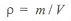where

m = Mass of the liquid, and
V = Volume of the liquid.

2. Weight density or specific weight.
It is defined as the weight per unit volume of a liquid at a standard temperature and pressure. It is usually denoted by w. It is expressed in kN/m3 or N/m3 or N/mm3. Mathematically, weight density or specific weight,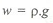Note : For water, w = 9.81 kN / m3 = 9.81 x 103 N / m3 = 9.81 x 10-6 N / mm3.

3. Specific volume. It is defined as the volume per unit mass of the liquid. It is denoted by v. Mathematically, specific volume,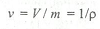4. Specific gravity. It is defined as the ratio of specific weight of a liquid to the specific weight of pure water at a standard temperature (4°C). It has no units.

Note: The specific gravity of pure water is taken as unity.

### Properties of Liquid

Viscosity, Kinematic viscosity, Compressibility, Surface tension and capillarity are most important properties of liquid. The following properties of liquid are important from the subject point of view

1. Viscosity. It is also known as absolute viscosity or dynamic viscosity. lt is defined as the property of a liquid which offers resistance to the movement of one layer of liquid over another adjacent layer of liquid. The viscosity of a liquid is due to cohesion and interaction between particles.

2. Kinematic viscosity. It is defined as the ratio of dynamic viscosity to the density of liquid.

3. Compressibility. It is that property of a liquid by virtue of which liquids undergo a change in volume with the change in pressure. The compressibility is the reciprocal of bulk modulus of elasticity, which is defined as the ratio of compressive stress to volumetric strain.

4. Surface tension. It is that property of a liquid which enables it to resist tensile stress. It is denoted by sigma. It is expressed in N / m.

5. Capillarity. It is defined as a phenomenon of rise or fall of a liquid surface in a small vertical tube held in a liquid relative to general level of the liquid. The height of rise or fall (h) in the tube is given by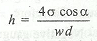where

sigma = Surface tension,
alpha = Angle of contact of the liquid surface,
w = Specific weight of liquid, and
d = Diameter of the capillary tube.

### Pressure of a Liquid

When a liquid is contained in a vessel, it exerts force at all points on the sides and bottom of the vessel. The force per unit area is called intensity of pressure. Mathematically, intensity of pressure,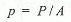where

P = Force acting on the liquid, and
A = Area on which the force acts.

The intensity of pressure at any point, in a liquid at rest is equal to the product of weight density of the liquid (w) and the vertical height from the free surface of the liquid (h) (it is also known as static head). Mathematically, intensity of pressure,

p = w.h

From this expression, we see that the intensity of pressure at any point, in a liquid, is directly proportional to depth of liquid from the surface.

The intensity of pressure may be expressed either in N / m2, N / mm2 or in metres of liquid or mm of liquid. It is also expressed in pascal (briefly written as Pa), such that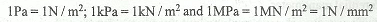### Pascal's Law

According to Pascal's law, the intensity of pressure at any point in a fluid at rest is same in all directions.

### Atmospheric Pressure, Gauge Pressure and Absolute Pressure

The atmospheric air exerts a normal pressure upon all surfaces with which it is in contact and it is known as atmospheric pressure. It is also known as barometric pressure. The atmospheric pressure at sea level (above absolute zero) is called standard atmospheric pressure and its value is given as follows :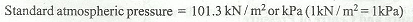= 10.3 m of water
= 760 mm of Hg

The pressure measured with the help of a pressure gauge is known as guage pressure, in which atmospheric pressure is taken as datum. All the pressure gauges record the difference between the actual pressure and the atmospheric pressure. The actual pressure is known as absolute pressure.

Mathematically

Absolute pressure = Atmospheric pressure + Gauge pressure (Positive)

This relation is used for pressures above atmospheric, i.e. for positive gauge pressure, as shown in Fig. 3.1 (a), For pressures below atmospheric, the gauge pressure will be negative. This negative gauge pressure is known as vacuum pressure. Therefore

Absolute pressure = Atmospheric pressure - Negative gauge pressure or vacuum pressure

This relation is shown in Fig 3.1 (b).